## 95. Examples using MPI_GATHER, MPI_GATHERVUp: Gather Next: Scatter Previous: Gather

The examples in this section use intracommunicators.
Example Gather 100 ints from every process in group to root. See figure 4 .

```    MPI_Comm comm;
int gsize,sendarray;
int root, *rbuf;
...
MPI_Comm_size( comm, &gsize);
rbuf = (int *)malloc(gsize*100*sizeof(int));
MPI_Gather( sendarray, 100, MPI_INT, rbuf, 100, MPI_INT, root, comm);
```

Example Previous example modified -- only the root allocates memory for the receive buffer.

```    MPI_Comm comm;
int gsize,sendarray;
int root, myrank, *rbuf;
...
MPI_Comm_rank( comm, &myrank);
if ( myrank == root) {
MPI_Comm_size( comm, &gsize);
rbuf = (int *)malloc(gsize*100*sizeof(int));
}
MPI_Gather( sendarray, 100, MPI_INT, rbuf, 100, MPI_INT, root, comm);
```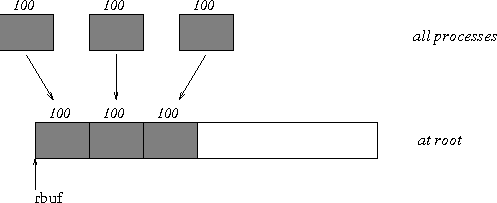Example Do the same as the previous example, but use a derived datatype. Note that the type cannot be the entire set of gsize*100 ints since type matching is defined pairwise between the root and each process in the gather.

```    MPI_Comm comm;
int gsize,sendarray;
int root, *rbuf;
MPI_Datatype rtype;
...
MPI_Comm_size( comm, &gsize);
MPI_Type_contiguous( 100, MPI_INT, &rtype );
MPI_Type_commit( &rtype );
rbuf = (int *)malloc(gsize*100*sizeof(int));
MPI_Gather( sendarray, 100, MPI_INT, rbuf, 1, rtype, root, comm);
```

Example Now have each process send 100 ints to root, but place each set (of 100) stride ints apart at receiving end. Use MPI_GATHERV and the displs argument to achieve this effect. Assume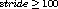. See Figure 5 .

```    MPI_Comm comm;
int gsize,sendarray;
int root, *rbuf, stride;
int *displs,i,*rcounts;

...

MPI_Comm_size( comm, &gsize);
rbuf = (int *)malloc(gsize*stride*sizeof(int));
displs = (int *)malloc(gsize*sizeof(int));
rcounts = (int *)malloc(gsize*sizeof(int));
for (i=0; i<gsize; ++i) {
displs[i] = i*stride;
rcounts[i] = 100;
}
MPI_Gatherv( sendarray, 100, MPI_INT, rbuf, rcounts, displs, MPI_INT,
root, comm);
```
Note that the program is erroneous if stride < 100.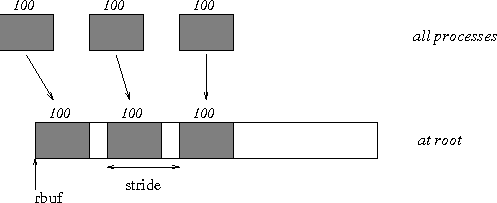Example Same as Example Examples using MPI_GATHER, MPI_GATHERV on the receiving side, but send the 100 ints from the 0th column of a 100×150 int array, in C. See Figure 6 .

```    MPI_Comm comm;
int gsize,sendarray;
int root, *rbuf, stride;
MPI_Datatype stype;
int *displs,i,*rcounts;

...

MPI_Comm_size( comm, &gsize);
rbuf = (int *)malloc(gsize*stride*sizeof(int));
displs = (int *)malloc(gsize*sizeof(int));
rcounts = (int *)malloc(gsize*sizeof(int));
for (i=0; i<gsize; ++i) {
displs[i] = i*stride;
rcounts[i] = 100;
}
/* Create datatype for 1 column of array
*/
MPI_Type_vector( 100, 1, 150, MPI_INT, &stype);
MPI_Type_commit( &stype );
MPI_Gatherv( sendarray, 1, stype, rbuf, rcounts, displs, MPI_INT,
root, comm);
```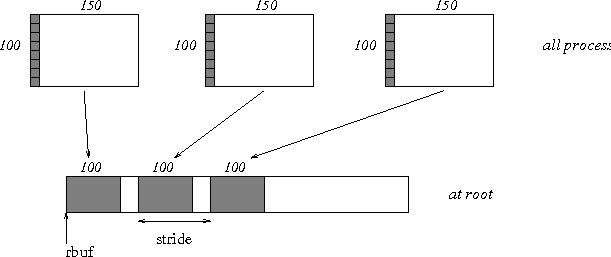Example Process i sends (100-i) ints from the i-th column of a 100 × 150 int array, in C. It is received into a buffer with stride, as in the previous two examples. See Figure 7 .

```    MPI_Comm comm;
int gsize,sendarray,*sptr;
int root, *rbuf, stride, myrank;
MPI_Datatype stype;
int *displs,i,*rcounts;

...

MPI_Comm_size( comm, &gsize);
MPI_Comm_rank( comm, &myrank );
rbuf = (int *)malloc(gsize*stride*sizeof(int));
displs = (int *)malloc(gsize*sizeof(int));
rcounts = (int *)malloc(gsize*sizeof(int));
for (i=0; i<gsize; ++i) {
displs[i] = i*stride;
rcounts[i] = 100-i;     /* note change from previous example */
}
/* Create datatype for the column we are sending
*/
MPI_Type_vector( 100-myrank, 1, 150, MPI_INT, &stype);
MPI_Type_commit( &stype );
/* sptr is the address of start of "myrank" column
*/
sptr = &sendarray[myrank];
MPI_Gatherv( sptr, 1, stype, rbuf, rcounts, displs, MPI_INT,
root, comm);
```
Note that a different amount of data is received from each process.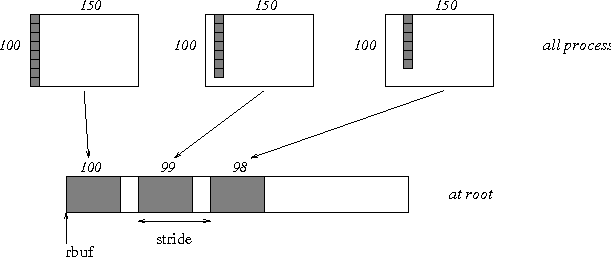Example Same as Example Examples using MPI_GATHER, MPI_GATHERV , but done in a different way at the sending end. We create a datatype that causes the correct striding at the sending end so that we read a column of a C array. A similar thing was done in Example Examples , Section Examples .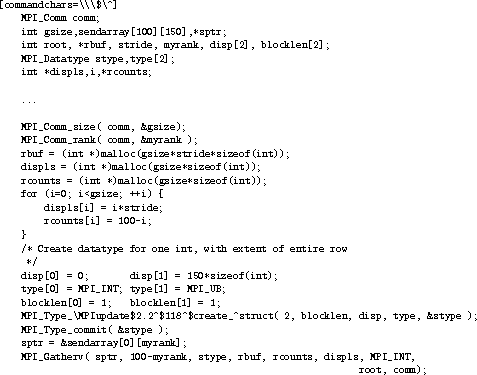Example Same as Example Examples using MPI_GATHER, MPI_GATHERV at sending side, but at receiving side we make the stride between received blocks vary from block to block. See Figure 8 .

```    MPI_Comm comm;
int gsize,sendarray,*sptr;
int root, *rbuf, *stride, myrank, bufsize;
MPI_Datatype stype;
int *displs,i,*rcounts,offset;

...

MPI_Comm_size( comm, &gsize);
MPI_Comm_rank( comm, &myrank );

stride = (int *)malloc(gsize*sizeof(int));
...
/* stride[i] for i = 0 to gsize-1 is set somehow
*/

/* set up displs and rcounts vectors first
*/
displs = (int *)malloc(gsize*sizeof(int));
rcounts = (int *)malloc(gsize*sizeof(int));
offset = 0;
for (i=0; i<gsize; ++i) {
displs[i] = offset;
offset += stride[i];
rcounts[i] = 100-i;
}
/* the required buffer size for rbuf is now easily obtained
*/
bufsize = displs[gsize-1]+rcounts[gsize-1];
rbuf = (int *)malloc(bufsize*sizeof(int));
/* Create datatype for the column we are sending
*/
MPI_Type_vector( 100-myrank, 1, 150, MPI_INT, &stype);
MPI_Type_commit( &stype );
sptr = &sendarray[myrank];
MPI_Gatherv( sptr, 1, stype, rbuf, rcounts, displs, MPI_INT,
root, comm);
```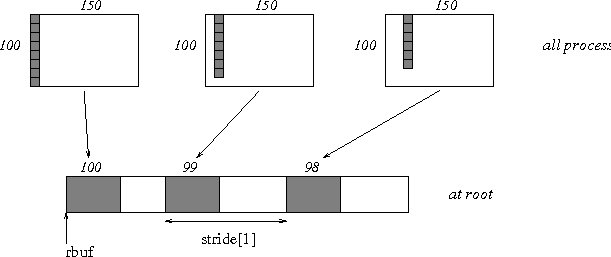Example Process i sends num ints from the i-th column of a 100 × 150 int array, in C. The complicating factor is that the various values of num are not known to root, so a separate gather must first be run to find these out. The data is placed contiguously at the receiving end.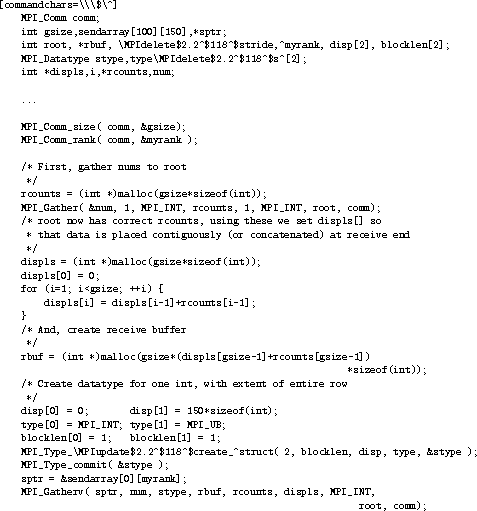Up: Gather Next: Scatter Previous: Gather